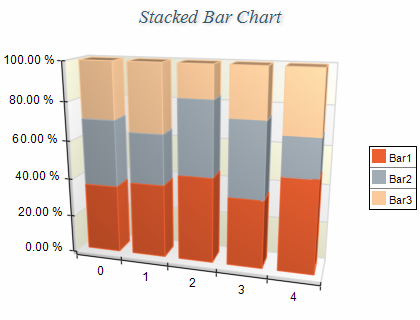﻿ Stacked Percent Bar
Nevron .NET Vision Documentation
Stacked Percent Bar

A 100% Stacked Bar chart displays all series stacked in a single column for each category. The height of each column is always the full height of the chart. The series values are displayed as percentages of each column. The Y scale range is always from 0 to 1 (0 - 100%). The following figure shows a typical Stack Percent Bar chart:Figure 1.

Creating the stacked bar chart

Stacked Percent Bar charts are displayed with several NBarSeries objects. The MultiBarMode property of the first bar series must be set to MultiBarMode.Series. The MultiBarMode property of the subsequent bar series must be set to MultiBarMode.StackedPercent. The following example demonstrates how to create a stacked percent bar chart with three stacks:

C#
Copy Code
```// obtain a reference to the Cartesian chart that is created by default
NCartesianChart chart = (NCartesianChart)chartControl.Charts;

bar1.MultiBarMode = MultiBarMode.Series;
bar2.MultiBarMode = MultiBarMode.StackedPercent;
bar3.MultiBarMode = MultiBarMode.StackedPercent;
```
Visual Basic
Copy Code
```' obtain a reference to the Cartesian chart that is created by default
Dim chart As NCartesianChart = chartControl.Charts(0)

Dim bar1 As NBarSeries = chart.Series.Add(SeriesType.Bar)
Dim bar2 As NBarSeries = chart.Series.Add(SeriesType.Bar)
Dim bar3 As NBarSeries = chart.Series.Add(SeriesType.Bar)

bar1.MultiBarMode = MultiBarMode.Series
bar2.MultiBarMode = MultiBarMode.StackedPercent
bar3.MultiBarMode = MultiBarMode.StackedPercent
```
Stacked Percent Scaling

When a bar series is displayed in stacked percent mode the min value of the series is always 0 and max value of the series is always 1. In order to display the texts on the PrimaryY axis formatted as percentage you have to use the following code:

C#
Copy Code
```NStandardScaleConfigurator sc = (NStandardScaleConfigurator)chart.Axis(StandardAxis.PrimaryY).ScaleConfigurator;
sc.LabelValueFormatter = new NNumericValueFormatter(NumericValueFormat.Percentage);
```
Visual Basic
Copy Code
```Dim sc As NStandardScaleConfigurator = chart.Axis(StandardAxis.PrimaryY).ScaleConfigurator
sc.LabelValueFormatter = New NNumericValueFormatter(NumericValueFormat.Percentage)
```
Stacks with negative values
Stacked percent bar charts do not support negative values, which means that all values are internally converted to their absolute values.
Formatting Commands

The following formatting commands inherited from the NSeries class have different meaning when a bar series is stacked:

<total> - displays the absolute sum of the values in the current stack.
<cumulative> - displays the sum of the values up to the current stack value.
<percent> - displays the percent contribution of the value to the total pile sum.

Related Examples
Windows forms: Chart Gallery\Bar\Stacked Bar